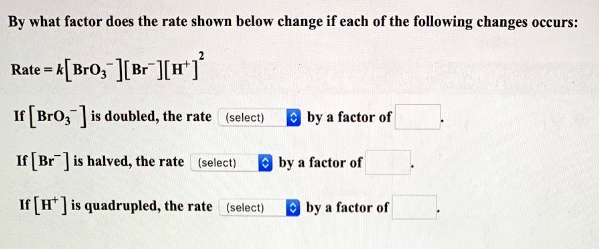# By what factor the rate shown below change if each of the following changes occurs: Rate = k[BrO3^-][Br^-][H^+]^2 If [BrO3^-] is doubled, the rate ____ by a factor of ____. If [Br^-] is halved, the rate ____ by a factor of ____. If [H^+] is quadrupled, the rate ____ by a factor of ___.